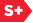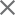### Proceedings Paper

Gabor transform: theory and computations
Author(s): Jie Yao
Format Member Price Non-Member Price
PDF \$17.00 \$21.00

Paper Abstract

In this paper, the theory and computations for the Gabor transform are discussed. The Gabor coefficients can be computed with a biorthogonal function or the Zak transform. Relations between a window function and its biorthogonal function are discussed. The formulas derived for the continuous variable Gabor transform with Zak transforms can be applied to the discrete Gabor transform by replacing the Zak transforms with the discrete Fourier transforms. The generalized Gabor transform are also discussed. Relations between a window function and its biorthogonal functions are presented. In the case of the generalized Gabor transform, the biorthogonal functions are not unique. The optimal biorthogonal functions are discussed. A relation between a window function and its optimal biorthogonal function is presented based on the Zak transform when T/T' is rational. The finite discrete generalized Gabor transform is also derived. The relations between a window function and its optimal biorthogonal function derived for the continuous variable generalized Gabor transform can be extended to the finite discrete case.

Paper Details

Date Published: 1 November 1993
PDF: 12 pages
Proc. SPIE 2034, Mathematical Imaging: Wavelet Applications in Signal and Image Processing, (1 November 1993); doi: 10.1117/12.162059
Show Author Affiliations
Jie Yao, Open Software Foundation (United States)

Published in SPIE Proceedings Vol. 2034:
Mathematical Imaging: Wavelet Applications in Signal and Image Processing
Andrew F. Laine, Editor(s)